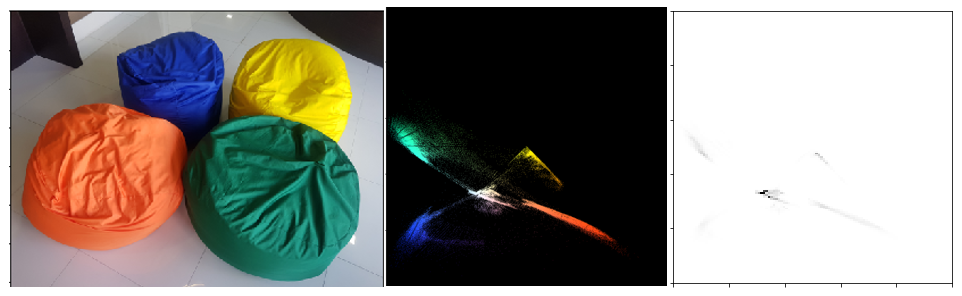# 10: Image (Chromaticity) Segmentation

Image segmentation using the different types of channels and thresholds may not work in cases where the wanted colors are not obvious. Instead, we look at the RG Chromaticity space to mask our image.

Chromaticity Segmentation relies on the RG Chromaticity space, a two-dimensional representation of color that removes information regarding the intensity value of the colors. We are looking at the proportion of the different color channels and map it using the normalized RGB space. The RG Chromaticity only have r and g because the b can be solved using the last equation.

To demonstrate the formula, let us consider 3 colors, pure red [255, 0, 0], a darker shade of red [128, 0, 0], and a mixed color [150, 50, 250]. The first 2 colors have the same values since

r = 255/(255+0+0) = 128/(128+0+0) = 1, g = 0, b = 0

For the last color, more calculations need to be done

r = 150/(150+50+250)=1/3, g = 1/9, b = 5/9

From these calculations, r and g can be plotted on the rg chromaticity space.RG Chromaticity Space & 2d Histogram Representation

Considering the image of the bean bags, running the calculations per color channel, masking the values onto the chromaticity space, and getting a 2d histogram, 5 main clusters of colors are visible.